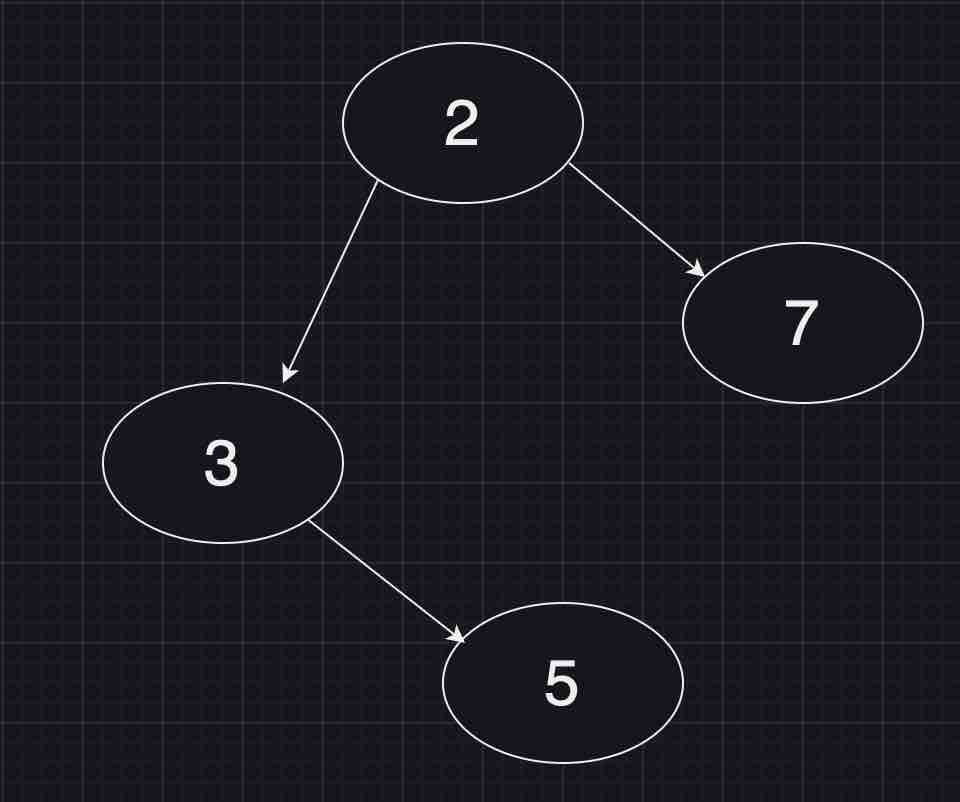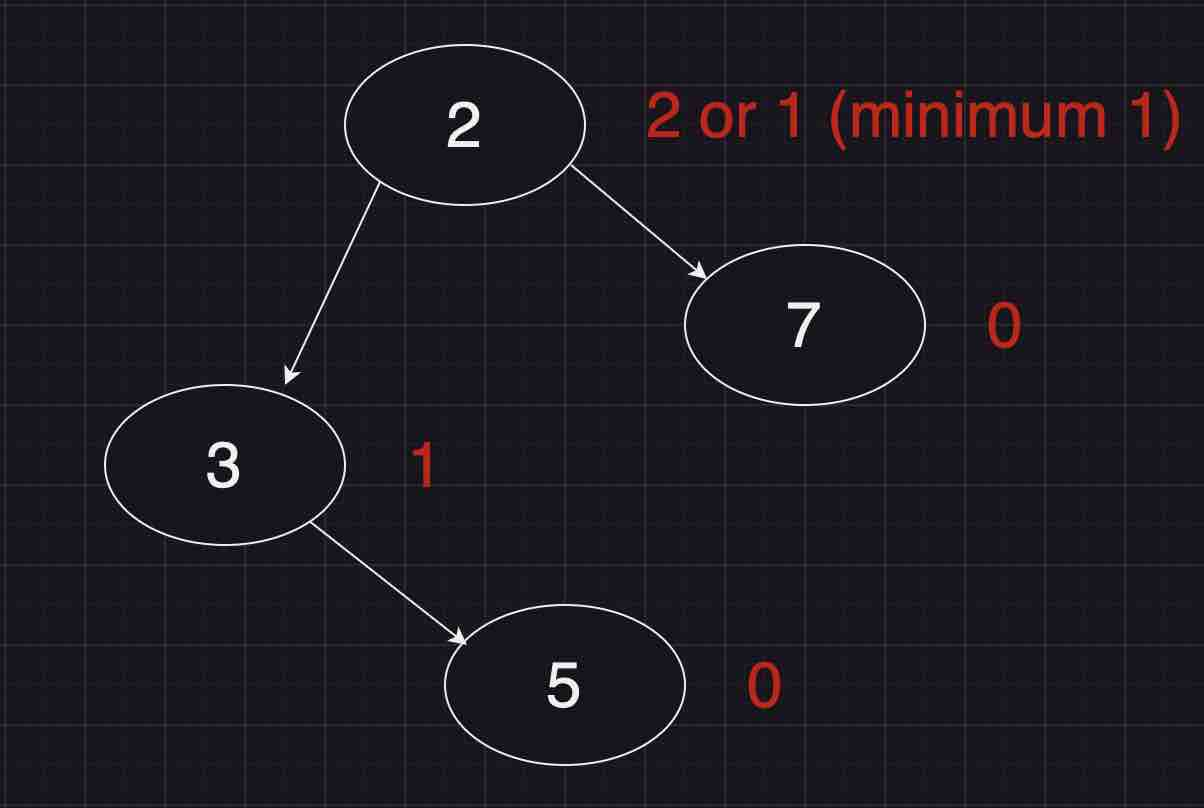Paul Coroneos
Published on

# Leetcode 111. Minimum Depth of Binary Tree

Today we will being going over Leetcode 111 - Minimum Depth of Binary Tree. This is a classic DFS (depth first search) problem we will implement using recursion.

Given a binary tree, find its minimum depth. The minimum depth is the number of nodes along the shortest path from the root node down to >the nearest leaf node.

Note: A leaf is a node with no children.

This may be a little cryptic without first examining an example of a tree. Lets take the below one for example:After analyzing the tree by inspection we see two possible paths:

• 2 -> 3 -> 5 (length 3)
• 2 -> 7 (length 2)

So how would we implement what we just did manually using the concept of recursion? Let's go through some of the typical considerations we would need to make when deciding to use recursion:

1. What is the base case?

What is the base case of this algorithm? Well it's when we reach a leaf node (a node with no left or right children). In this case there is no additional length so we would return zero.

1. What is the recursive case?

Well we have three possible sub scenarios. Let's take them one at a time

There is a left child node but no right child node

If there is a left child node but no right child node there's really no reason to recurse down the right node. After all when we call our recursive function on this node (node.right) per our base case we will reutrn zero. So just recursing down the path of the left child is acceptable.

There is a right child node but no left child node

This case is basically the same as the previous case just the other way around

There are both left and right child nodes

This case is pretty straightforward but let's talk through what the expectation is. As we continue to recurse down the tree (we are doing DFS here) we will keep putting calls on the stack until we hit all leaf nodes in the tree. As we then start to pop off function calls after resolving the nodes in the tree bottom up we learn something each time.

The learning each time as we climb up the tree is what the length of the path is so far up until that given node. A little confusing? Let's take a look at the tree diagram again except with some annotations this time:Imagine starting at the bottom of the tree and working your way up. As you can see as we go backwards from the leaf nodes back up to the root each node along the way gains knowledge from its children in that we determine the minimum length of the path so far. This eloquently allows us to use DFS to solve this problem.

So now why did we go down this tangent? Well in the case of a left and right child node we have a decision to make. Which path length is the length we want to take? Given this problem asks us what the minimum path length is we therefore take the minimum of the two paths. Or in other words we really dont care anything about what has happened so far for any arbitrary pathing below this node. We just want to take whichever path is the shortest.

So now that we have a good understanding of the problem let's take a look at the code:

``````/**
* Definition for a binary tree node.
* function TreeNode(val, left, right) {
*     this.val = (val===undefined ? 0 : val)
*     this.left = (left===undefined ? null : left)
*     this.right = (right===undefined ? null : right)
* }
*/
/**
* @param {TreeNode} root
* @return {number}
*/
var minDepth = function (root) {
if (!root) return 0;
if (!root.left) return minDepth(root?.right) + 1;
if (!root.right) return minDepth(root?.left) + 1;

return Math.min(minDepth(root.left), minDepth(root.right)) + 1;
};
``````

So this would follow what we outlined above pretty well. But the question may arise why do we add the value of "one" to the return of all the recursive calls. The answer is quite simply _we need to take into account the fact we have visited a node and thus add it to our total path length. Or again in other words the recursive calls are actually incrementing our minimum path length by one each time we decide to "pick" a path and continue climbing the tree to the root node; When we have run out of nodes to iterate through we have returned the minimum length.

Thank you so much for your time reading this and best of luck on your journey learning algorithms!